# KSSEB Solutions for Class 9 Maths Chapter 11 Areas of Parallelograms and Triangles Ex 11.4

## Karnataka Board Class 9 Maths Chapter 11 Areas of Parallelograms and Triangles Ex 11.4

Question 1.
Parallelogram ABCD and rectangle ABEF are on the same base AB and have equal areas. Show that the perimeter of the parallelogram is greater than that of the rectangle.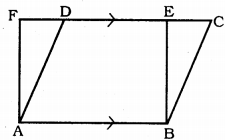Solution:
Data: Parallelogram ABCD and rectangle ABEF are on the same base AB and have equal areas.
To Prove: Perimeter of the parallelogram is greater than that of the rectangle.
Proof: ||grm ABCD and rectangle ABEF are on the same base AB and in between AB || FC.
AB = CD (Opposite sides of ||grm)
AB = EF (Opposite side of rectangle)
∴ CD = EF
∴ AB + CD = AB + EF …………. (i)
Perpendicular drawn from the vertex to base is only smaller than the remaining lines.
∴ FA < AD and BE < BC
∴ AF + BE < AD + BC ………. (ii)
Comparing (i) and (ii),
(AB + CD + AF + BE) < (AB + EF + AD + BC)
∴ Perimeter of the parallelogram is greater than that of the rectangle.

Question 2.
In Fig., D and E are two points on BC such that BD = DE = EC. Show that ar.(ABD) = ar.(ADE) = ar.(AEC).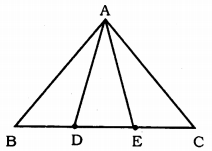Can you now answer the question that you have left in the ‘Introduction’ of this chapter, whether the field of Budhia has been actually divided into three parts of equal area?
Solution:
Data : D and E are two points on BC such that BD = DE = EC.
To Prove: ar.(ABD) = ar.(ADE) = ar.(AEC)
Construction: Draw AM ⊥ BC.[Remark: Note that by taking BD = DE = EC, the triangle ABC is divided into three triangles ABD,- ADE, and AEC of equal areas. In the same way, by dividing BC into ‘n’ equal parts and joining the points of division so obtained to the opposite vertex of BC, you can divide AABC into ‘n’ triangles of equal areas.]

Question 3.
In Fig., ABCD, DCEF and ABFE are parallelograms. Show that ar. (∆ADE) = ar. (∆BCF).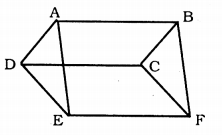Solution:
Data: ABCD, DCEF, and ABFE are Parallelograms.
Proof: In ||grm ABCD,
∴ AD = BC (Opposite sides of ||grm) ………… (i)
Similarly, in DCFE,
DE = CF (Opposite sides of ||grm) ………..(ii)
Similarly, in ABFE,
AE = BF (Opposite sides of ||grm) ………… (iii)
DE = CF ……… (2)
AE = BF ……… (3)
∴ ∆ADE ≅ ∆BCF (SSS postulate)

Question 4.
In Fig., ABCD is a parallelogram, and BC is produced to a point Q such that AD = CQ. If AQ intersect DC at P, show that ar(∆BPC) = ar(∆DPQ).
(Hint: Join AC).Solution:
Data: ABCD is a parallelogram and BC is produced to a point Q such that AD = CQ. AQ intersect DC at P,
To Prove: ar.(∆BPC) = ar.(∆DPQ)
Construction: AE is joined.
Proof: ABCD is a parallelogram.
∆APC and ∆BDC are on BC and in between AB || PC.
∴ ∆APC = ∆BPC …………… (i)
∴ ∆BCD is a parallelogram.
∆DQC and ∆AQC are on base QC and in between QC || AD.
∴ ∆DQC = ∆AQC
ar.(∆DQC) – ar.(∆PQC) = ar.(∆ACQ) – ar.(∆PQC)
∴ ar.(∆APC) = ar.(∆DPQ) ………….. (ii)
Comparing (i) and (ii),
ar.(∆BPC) = ar.(∆DPQ).

Question 5.
In Fig., ∆ABC and ∆BDE are two equilateral triangles such that D is the mid-point of BC. If AE intersects BC at F, show that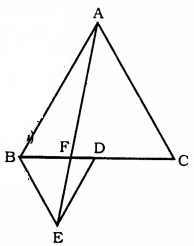(i) ar.(∆BDE) = $$\frac{1}{4}$$ ar.(∆ABC)
(ii) ar.(∆BDE) = $$\frac{1}{2}$$ ar.(∆BAE)
(iii) ar.(∆ABC) = 2ar.(∆BEC)
(iv) ar.(∆BFE) = ar.(∆AFD
(v) ar.(∆BFE) = 2ar.(∆FE:
(vi) ar.(∆FED)= $$\frac{1}{8}$$ ar.(∆AFC)
[Hint: Join EC and AD. Show that BE || AC and DE || AB, etc.]
Solution:
Data : ∆ABC and ∆BDE are two equilateral triangles such that D is the mid-point of BC. AE intersects BC at F,
To Prove:
(i) ar.(∆BDE) = $$\frac{1}{4}$$ ar.(∆ABC)
(ii) ar.(∆BDE) = $$\frac{1}{2}$$ ar.(∆BAE)
(iii) ar.(∆ABC) = 2ar.(∆BEC)
(iv) ar.(∆BFE) = ar.(∆AFD
(v) ar.(∆BFE) = 2ar.(∆FE:
(vi) ar.(∆FED)= $$\frac{1}{8}$$ ar.(∆AFC)
Construction: BC and AD are joined. G and H are midpoints of AB and AC resply. GH, GD and HD are joined.
Proof: (i) D is the midpoint of BC.
Now, GH || BC OR GH || BD (Data)
GH = $$\frac{1}{2}$$ BC OR GH = BD
∴ BDHG is a parallelogram.
∴ ar.(∆GHD) = ar. (∆BDG)
Similarly, GDCH is a parallelogrm.
∴ ar.(∆GHD) = ar.(∆DCH)
AGDH is a parllelogrm.
∴ ar.(∆GHD) = ar.(∆AGH)
∆ABC = ∆BDG + ∆GHD + ∆AGH + ∆HDC
∴ ∆ABC = 4 × ∆BDE
∴ ar.(∆BDE) = $$\frac{1}{4}$$ × ar.(∆ABC).

(ii) ∆BDE = ∆AED
(∆BDE) – (∆FED) = (∆AED) – (∆FED)
∴ (∆BEF) = (∆ADF) ………. (i)
(∆ABD) = (∆ABF) + (∆BEF) [from (i)]
∴ (∆ABD) = (∆ABE) …………. (ii)
But, (∆ABD) = $$\frac{1}{2}$$ × (∆ABC)
(∆ABD) = $$\frac{1}{2}$$ × 4W(∆BDE)
∴ ar.(∆ABD) = 2 × ar.(∆BDE) ………… (iii)
From (i) and (ii),
ar.(∆ABE) = 2 × ar.(∆BDE)
∴ ar.(∆BED) = $$\frac{1}{2}$$ × ar.(∆ABE)

(iii) ∆ABE and ∆BEC are on same base BE and in between AC || BE.
∴ (∆ABE) = (∆BEC)
But, ar.(∆ABE) = 2 × ar.(∆BDE) (proved)
∴ 2ar.(∆BDE) = ar.(∆BEC)
But, ar.(∆BDE) = $$\frac{1}{4}$$ × ar.(∆ABC)
∴ 2 × $$\frac{1}{4}$$ ar.(∆ABC) = ar.(∆BEC)
∴ r.(∆BEC) = $$\frac{1}{2}$$ × ar.(∆ABC)
ar.(∆ABC) = 2 × ar.(∆BEC)

(iv) ∆BED and ∆AED are on same base DE and in between AB || DE.
∴ ar. (∆BED) = ar.(∆AED)
ar.(∆BED) – ar.(∆DEF) = ar.(∆AED) – ar.(∆DEF)
ar.(∆BEF) = ar.(∆AFD)

(v) Let height of ∆ABC be ‘a’ and height of DBDE be V.= ar. (∆BEF) + $$\frac{1}{2}$$ ar.(∆ABC)
= ar.(∆BEF) + $$\frac{1}{2}$$ × 4ar.(∆BED)
= ar.(∆BFE) + 2ar.(∆BDE)
But, ar.(∆BED) = ar.(∆BFE) + ar.(∆FED)
= 2 × ar.(∆FED) + ar.(∆FED)
= 3 × ar.(∆FED)
∴ ar.(∆AFC) = 2 × ar.(∆FED) + 2 × 3 ar.(∆FED)
= 2 × ar.(∆FED) + 6 ×ar.(∆FED)
ar.(∆AFC)= 8 × ar.(∆FED)
ar.(∆FED) = $$\frac{1}{8}$$ × ar.(∆AFC)

Question 6.
Diagonals AC and BD of a quadrilateral ABCD intersect each other at P. Show that
ar.(∆APB) × ar.(∆CPD) = ar.(∆APD) × ar.(∆BPC)
[Hint: From A and C, draw perpendiculars to BD).Solution:
Data: Diagonals AC and BD of a quadrilateral ABCD intersect each other at P.
To Prove: ar.(∆APB) × ar.(∆CPD) = ar.(∆APD) × ar.(∆BPC)
Construction: Draw AM ⊥ DB, CN ⊥ DB.
Proof: ar.(∆APB) × ar.(∆CPD) =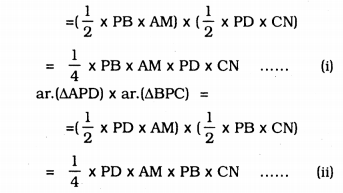From (i) and (ii), .
ar.(∆APB) × ar.(∆CPD) = ar.(∆APD) × ar.(∆BPC).

Question 7.
P and Q are respectively the mid-points of sides AB and BC of a triangle AABC and R is the mid-point of AP, Show that(i) ar.(∆PRQ) = $$\frac{1}{2}$$ ar.(∆ARC)
(ii) ar.(∆RQC) = $$\frac{3}{8}$$ ar.(∆ABC)
(iii) ar.(∆PBQ) = ar.(∆ARC).
Solution:
Data : P and Q are respectively the mid-points of sides AB and BC of a triangle ∆ABC and R is the mid-point of AP.
To Prove:
(i) ar.(∆PRQ) = $$\frac{1}{2}$$ ar.(∆ARC)
(ii) ar.(∆RQC) = $$\frac{3}{8}$$ ar.(∆ABC)
(iii) ar.(∆PBQ) = ar.(∆ARC).
Proof: P and Q are respectively the mid points of sides aB and BD
∴ PQ || AC and PQ = $$\frac{1}{2}$$ AC
(∵ Mid-point theorem)
Mark S such that it is the mid point of AC and join QS.
∴ PQSA is a parallelogram. Its diagonals bisect quadrilateral into two congruent triangles.
ar.(∆PAS) = ar.(∆PQS) = ar.(∆PQA) = ar.(∆QAS)
Similarly, PSCQ, QSCT and PSQB are parallelograms.
∴ ar. (∆PSQ) = ar.(∆CQS)
ar.(∆QSC)= ar.(∆CTQ)
ar. (∆PSQ)=ar. (∆QBP)
∴ ∆PAS = ∆SQP = ∆PAQ = ∆SQA = ∆QSC = ∆CTQ = ∆QBP …………… (i)
and ∆ABC = ∆PBQ + ∆PAS + ∆PQS + ∆QSC
∆ABC = ∆PBQ + ∆PBQ + ∆PBQ + ∆PBQ
∆ABC = 4 × ∆PBQ
ar.(∆ABC) = 4 × ar.(∆PBQ)
ar.(∆PBQ) = $$\frac{1}{4}$$ × ar.(∆ABC) …………….. (ii)

(i) P and C are joined.
QR is the median of ∆PAQ.
∴ ar.(∆PQR) = ar.(∆AQR)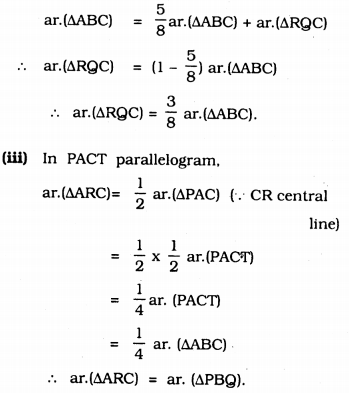Question 8.
In Fig., ABC is a right triangle right angled at A, BCED, ACFG and ABMN are squares on the sides BC, CA and AB respectively. Line segment AX ⊥ DE meets BC at Y. Show that :
(i) ∆MBC ≅ ∆ABD
(ii) ar.(BYXD) = 2ar.(∆MBC)
(iii) ar.(BYXD) = ar.(ABMN)
(iv) ∆FCB ≅ ∆ACE
(v) ar.(CYXE) = 2ar.(∆FCB)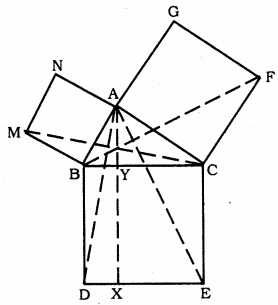(vi) ar.(CYXE) = ar,(ACFG)
(vii) ar.(BCED) = ar.(ABMN) + ar.(ACFG).
Solution:
Data: ABC is a right-angled triangled at A, BCED, ACFG and ABMN are squares on the sides BC, CA and AB respectively. Line segment AX ⊥ DE meets BC at Y.To Prove:
(i) ∆MBC ≅ ∆ABD
(ii) ar.(BYXD) = 2ar.(∆MBC)
(iii) ar.(BYXD) = ar.(ABMN)
(iv) ∆FCB ≅ ∆ACE
(v) ar.(CYXE) = 2ar.(∆FCB)
(vi) ar.(CYXE) = ar.(ACFG)
(vii) ar.(BCED) = ar.(ABMN) + ar.(ACFG).
Proof: (i) Each angle of the square is 90°.
∴ ∠ABM = ∠DBC = 90°
∠ABM + ∠ABC = ∠DBC + ∠ABC
∴ ∠MBC = ∠ABD
In ∆MBC and ∆ABD,
∠MBC = ∠ABD (Proved)
MB = AB
∴ ∆MBC ≅ ∆ABD (ASA Postulate)

(ii) ∆MBC = ∆ABD
∴ ar.(∆MBC) = ar.(∆ABD) ………… (i)
AX ⊥ DE, BD ⊥ DE
∴ BD || AX
∆ABD and quadrilateral BYXD are on base BD and in between BD || AX.
ar.(∆ABD) = $$\frac{1}{2}$$ × ar.(BYXD)
BYXD = 2 × ar.(∆ABC)
BYXD = 2 × ar.(∆MBC) …………(ii)

(iii) ∆MBC, quadrilateral ABMN are on base MB and in between MB || NC.
∴ ar.(∆MBC) = $$\frac{1}{2}$$ × ar.(ABMN)
2 × ar.(∆MBC) = ar.(ABMN)
ar.(BYXD) = ar.(ABMN) ……………….. (iii)

(iv) Each angle of square is 90°.
∴ ∠FCA = ∠BCE = 90°
∠FCA + ∠ACB = ∠BCE + ∠ACB
∴ ∠FCB = ∠ACE
In ∆FCB and ∆ACE,
∠FCB = ∠ACE
FC = AC
CB = CE
∴ ∆FCB ≅ ∆ACE (SAS postulte)

(v) AX ⊥ DE, CE ⊥ DE (Data)
∴ CE || AX
∆ACE, quadrilateral CYXE are on base CE and in between CE || AX.
∴ ar.(∆ACE) = $$\frac{1}{2}$$ ar.(CYXE)
ar.(CYXE) = 2 × ∆ACE …………… (iv)
∆FCB ≅ ∆ACE Proved.
∴ W(∆FCB) = W(∆ACE) …………… (v)
Comparing (iv) and (v),
ar.(CYXE) = 2 × ar.(∆FCB) ……………. (vi)

(vi) ∆FCB, and quadrilateral ACFG are on base CF and in between CF || BG.
∴ ar.(∆FCB) = $$\frac{1}{2}$$ × ar.(∆CFG)
ar.(ACFG) = 2 × ar.(∆FCB) …………… (vii)

(vii) ar.(BCED) = ar.(BYXD) + ar.(CYXE)
∴ ar.(BCED) = ar.(ABMN) + ar.(ACFG).
[Note: Result (vii) is the famous Theorem of Pythagoras. You shall learn a simpler proof of this theorem in Class X.]

##### KSEEB Solutions for Class 9 Maths
error: Content is protected !!